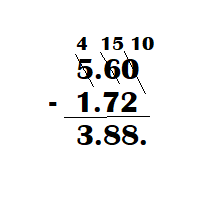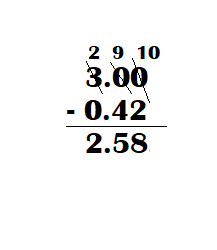# Math in Focus Grade 4 Chapter 8 Practice 3 Answer Key Subtracting Decimals

Practice the problems of Math in Focus Grade 4 Workbook Answer Key Chapter 8 Practice 3 Subtracting Decimals to score better marks in the exam.

## Math in Focus Grade 4 Chapter 8 Practice 3 Answer Key Subtracting Decimals

Fill in the blanks. Write each difference as a decimal.

Example
0.9 – 0.4 = 9 tenths – 4 tenths
= 5 tenths
= 0.5

Question 1.
1 – 0.3 = ___ tenths – ___ tenths
= ___ tenths
= ____
1 – 0.3 = 0.7.

Explanation:
1 – 0.3 = 10 tenths – 3 tenths
= 7 tenths
= 0.7.

Question 2.
1.3 – 0.6 = ___ tenths – ___ tenths
= ___ tenths
= ____
1.3 – 0.6 = 0.7.

Explanation:
1.3 – 0.6 = 13 tenths – 6 tenths
= 7 tenths
= 0.7.

Question 3.
1.8 – 0.9 = ___ tenths – ___ tenths
= ___ tenths
= ____
1.8 – 0.9 = ___ tenths – ___ tenths
= ___ tenths
= ____

Explanation:
1.8 – 0.9 = 18 tenths – 9 tenths
= 9 tenths
= 0.9.

Fill in the blanks.
Question 4.
Step 1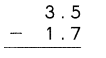You cannot subtract 7 tenths from 5 tenths. So, regroup 3 ones and 5 tenths. 3 ones and 5 tenths
= ___ ones and ___ tenths
Subtract the tenths.
___ tenths – 7 tenths = ____ tenths
Step 2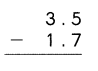Subtract the ones.
___ ones – ___one
= ___ one
So, 3.5 – 1.7 = ____
3.5 – 1.7 = 1.8.

Explanation:
Step 1 :You cannot subtract 7 tenths from 5 tenths. So, regroup 3 ones and 5 tenths. 3 ones and 5 tenths
= 2 ones and 15 tenths
Subtract the tenths.
15 tenths – 7 tenths = 8 tenths.
Step 2 :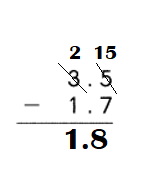Subtract the ones.
2 ones – 1 one
= 1 one.
So, 3.5 – 1.7 = 1.8.

Subtract.
Question 5.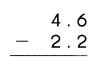4.6 – 2.2 = 2.4.

Explanation:
Step 1 :Subtract the tenths :
6 tenths – 2 tenths = 4 tenths.

Step 2 :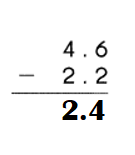Subtract the ones.
4 ones – 2 ones
= 2 ones.
So, 4.6 – 2.2 = 2.4.

Question 6.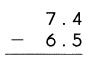7.4 – 6.5 = 0.9.

Explanation:
Step 1 :You cannot subtract 5 tenths from 4 tenths. So, regroup 7 ones and 4 tenths. 7 ones and 4 tenths
= 6 ones and 14 tenths.
Subtract the tenths.
14 tenths – 5 tenths = 9 tenths.
Step 2 :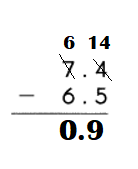Subtract the ones.
6 ones – 6 ones
= 0 ones.
So, 7.4 – 6.5 = 0.9.

Write in vertical form. Then subtract.
Question 7.
6.7 – 2.4 = ___
6.7 – 2.4 = 4.3.

Explanation: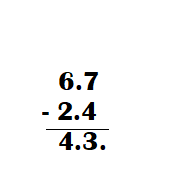Question 8.
3 – 1.3 = ____
3 – 1.3 = 1.7.

Explanation: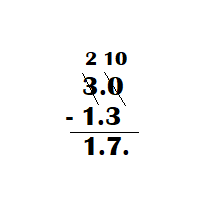Fill in the blanks. Write each difference as a decimal.
Example
0.08 – 0.02 = 8 hundredths – 2 hundredths
= 6 hundredths
= 0.06

Question 9.
0.23 – 0.19 = ___ hundredths – ___ hundredths
= ___ hundredths
= ____
0.23 – 0.19 = 0.04.

Explanation:
0.23 – 0.19 = 23 hundredths – 19 hundredths
= 4 hundredths
= 0.04.

Question 10.
0.1 – 0.06 = ___ hundredths – ___ hundredths
= ___ hundredths
= ____
0.1 – 0.06 = 0.94.

Explanation:
0.1 – 0.06 = 100 hundredths – 6 hundredths
= 94 hundredths
= 0.94.

Fill in the blanks.
Question 11.
Step 1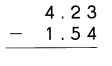You cannot subtract 4 hundredths from 3 hundredths. So, regroup 2 tenths 3 hundredths.
2 tenths 3 hundredths
= ___ tenth ___ hundredths
Subtract the hundredths.
___ hundredths – ___ hundredths
= ___ hundredths
Step 2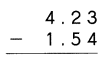You cannot subtract 5 tenths from ___ tenth.
So, regroup 4 ones and ___ tenth.
4 ones and ___ tenth
= ___ ones and ___ tenths
Subtract the tenths.
___ tenths – 5 tenths = ___ tenths
Step 3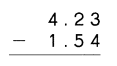Subtract the ones.
___ ones – 1 one = ___ ones
So, 4.23 – 1.54 = ___

4.23 – 1.54 = 2.69.

Explanation:
Step 1:You cannot subtract 4 hundredths from 3 hundredths. So, regroup 2 tenths 3 hundredths.
2 tenths 3 hundredths
= 1 tenth 13 hundredths
Subtract the hundredths.
13 hundredths – 4 hundredths
= 9 hundredths.
Step 2:You cannot subtract 5 tenths from 1 tenth.
So, regroup 4 ones and 1 tenth.
3 ones and 11 tenth
= 4 ones and 11 tenths
Subtract the tenths.
11 tenths – 5 tenths = 6 tenths.
Step 3:Subtract the ones.
3 ones – 1 one = 2 ones.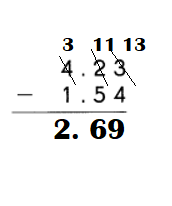Subtract.
Question 12.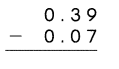0.39 – 0.07 = 0.32.

Explanation:
Step 1:Subtract the hundredths.
9 hundredths – 7 hundredths
= 2 hundredths.
Step 2:Subtract the tenths.
3 tenths – 0 tenths = 3 tenths.
Step 3:Subtract the ones.
0 ones – 0 one = 0 ones.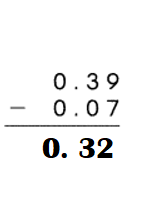Question 13.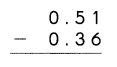0.51 – 0.36 = 0.15.

Explanation:
Step 1You cannot subtract 6 hundredths from 1 hundredths. So, regroup 5 tenths 1 hundredths.
5 tenths 1 hundredths
= 5 tenth 1 hundredths.
Subtract the hundredths.
11 hundredths – 6 hundredths
= 5 hundredths.
Step 2:Subtract the tenths.
4 tenths – 3 tenths = 2 tenths.
Step 3:Subtract the ones.
0 ones – 0 one = 0 ones.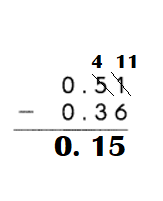Question 14.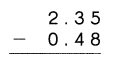2.35 – 0.48 = 1.87.

Explanation:
Step 1:You cannot subtract 8 hundredths from 5 hundredths. So, regroup 3 tenths 5 hundredths.
= 2 tenth 15 hundredths
Subtract the hundredths.
15 hundredths – 8 hundredths
= 7 hundredths.
Step 2:You cannot subtract 4 tenths from 2 tenth.
So, regroup 2 ones and 2 tenth.
1 ones and  12 tenth.
Subtract the tenths.
12 tenths – 4 tenths = 8 tenths.
Step 3:Subtract the ones.
1 ones – 0 one = 1 ones.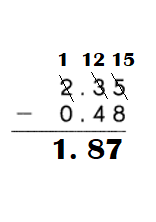Question 15.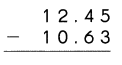12.45 – 10.63 = 1.82.

Explanation:
Step 1:Subtract the hundredths.
5 hundredths – 3 hundredths
= 2 hundredths.
Step 2:You cannot subtract 6 tenths from 4 tenth.
So, regroup 2 ones and 4 tenth.
= 1 ones and 14 tenths
Subtract the tenths.
14 tenths – 6 tenths = 8 tenths.
Step 3:Subtract the ones.
1 ones – 0 one = 1 ones.
Step 4:
Subtract the tens.
1 tens – 1 tens = 0 tens.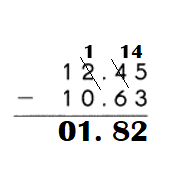Question 16.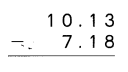10.13 – 7.18 = 2.95.

Explanation:
Step 1:You cannot subtract 8 hundredths from 3 hundredths. So, regroup 1 tenths 3 hundredths.
0 tenths 13 hundredths.
Subtract the hundredths.
13 hundredths – 8 hundredths
= 5 hundredths.
Step 2:You cannot subtract 1 tenths from 0 tenth.
So, regroup 0 ones and 0 tenth.
regroup 1 tens 0 tenths.
9 ones and 10 tenth.
= 9 ones and 10 tenths
Subtract the tenths.
10 tenths – 1 tenths = 9 tenths.
Step 3:Subtract the ones.
9 ones – 7 one = 2 ones.
So, 4.23 – 1.54 = ___
Step 4:Subtract the tens.
0 tens – 0 tens = 0 tens.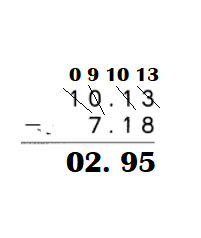Question 17.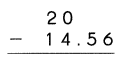20.00 – 14.56 = 5.44.

Explanation:
Step 1:You cannot subtract 6  hundredths from 0 hundredths. So, regroup 0 tenths 0 hundredths. So, regroup 0 ones 0 hundredths. So, regroup 2 tens 0 ones.
So, 1 tens. So, 10 ones. So, 10 tenths. So, 10 hundredths.
9 tenths 10 hundredths
= 9 tenth 10 hundredths.
Subtract the hundredths.
10 hundredths – 6 hundredths
= 4 hundredths
Step 2:Subtract the tenths.
9 tenths – 5 tenths = 4 tenths.
Step 3:Subtract the ones.
9 ones – 4 one = 5 ones.
Step 4:Subtract the tens .
1 ones – 1 one = 0 ones.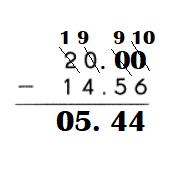Write in vertical form. Then subtract.
Question 18.
5.38 – 2.73 = ____
5.38 – 2.73 = 2.65.

Explanation: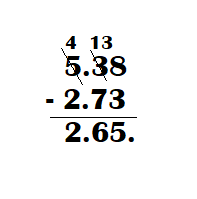Question 19.
1.06 – 0.38 = ___
1.06 – 0.38 = 0.68.

Explanation: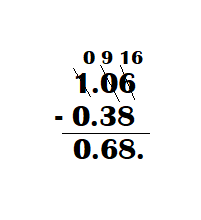Question 20.
5.6 – 1.72 = ___